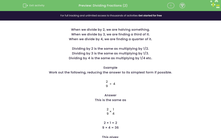# Dividing Fractions (2)

In this worksheet, students divide proper fractions by whole numbers, writing the answer in its simplest form.Key stage:  KS 2

Curriculum topic:   Number: Fractions, Decimals and Percentages

Curriculum subtopic:   Divide Proper Fractions

Difficulty level:#### Worksheet Overview

When we divide by 2, we are halving something.

When we divide by 3, we are finding a third of it.

When we divide by 4, we are finding a quarter of it.

Dividing by 2 is the same as multiplying by 1/2.

Dividing by 3 is the same as multiplying by 1/3.

Dividing by 4 is the same as multiplying by 1/4 etc.

Example

Work out the following, reducing the answer to its simplest form if possible.

 2 ÷ 4 9

This is the same as

 2 × 1 9 4

2 × 1 = 2

9 × 4 = 36

This gives:

 2 36

But this must be reduced by dividing top (numerator) and bottom (denominator) by 2.

 1 18

which we write as   1/18.

Want a bit more help with this before you begin? Why not watch this short video?

### What is EdPlace?

We're your National Curriculum aligned online education content provider helping each child succeed in English, maths and science from year 1 to GCSE. With an EdPlace account you’ll be able to track and measure progress, helping each child achieve their best. We build confidence and attainment by personalising each child’s learning at a level that suits them.

Get started